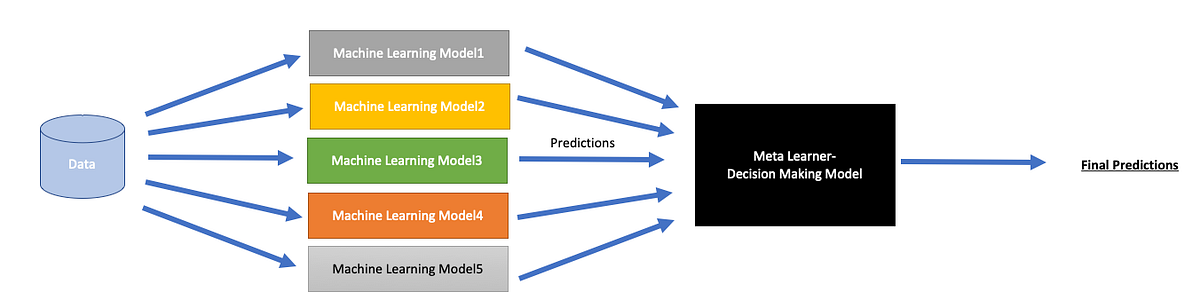# Ensemble models for ClassificationThis post was originally published by Gaurika Tyagi at Towards Data ScienceAn algorithm that is used to combine the base estimators is called the meta learner. We can determine how we want this algorithm to respond to different predictions from other models(classifiers in this case). It can be:

1. Predictions of estimators
2. Predictions as well as the original training data

But is it the final class predictions only? No, you can choose what metric drives that decision:

1. It could be `'predict_proba'`, or `'predict'` or both
2. You can also use other `'decision_function'` in sklearn

The final meta learner can then be any of the estimators (by default sklearn has logistic regression)

We take a baseline Gaussian Naive Bayes estimator and compare all future results to its prediction accuracy:

```rf = OneVsRestClassifier(estimator = GaussianNB())cv = RepeatedStratifiedKFold(n_splits=10, n_repeats=3, random_state=20)n_scores = cross_val_score(rf, X, y, scoring='f1_weighted', cv=cv, error_score='raise')print(n_scores)
print('Baseline scores n mean f1 weighted: %.3f with a %.3f standard deviation in scores ' % (np.mean(n_scores), np.std(n_scores)))
```

Image by Author: Base Model Scores

Create layers of tuned estimators and stack them together

Image by Author: Stacking model Outperformed other models!

Image by Author: Stacking model outperformed all Classifiers

In the end, we wanted a classifier with an F1 score higher than 81.1% and we ended up getting an F1 score of 96.7% with a stacking model.

In the healthcare setup, I will take that 0.1% improvement over SVC models and not trade-off the stacked model’s complexity!

You can find the full code here: https://github.com/gaurikatyagi/Machine-Learning/blob/master/Classification/Ensemble%20Model-%20Stacked%20Classification.ipynb

Go stack now…